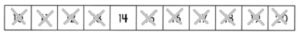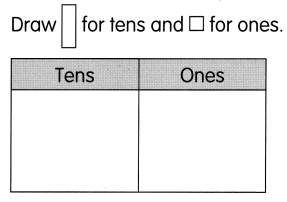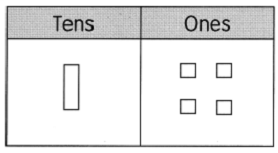# Math in Focus Grade 1 Chapter 7 Answer Key Numbers to 20

This handy Math in Focus Grade 1 Workbook Answer Key Chapter 7 Numbers to 20 detailed solutions for the textbook questions.

## Math in Focus Grade 1 Chapter 7 Answer Key Numbers to 20

Count how many stickers the Fill in the blanks.

Question 1.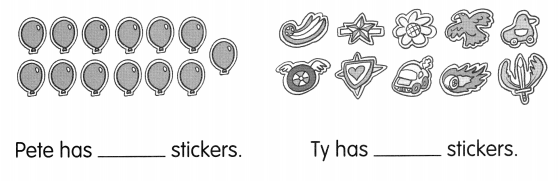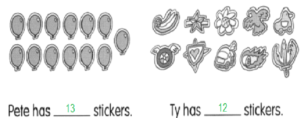Draw how many stickers you have. Then fill in the blanks.

Question 2.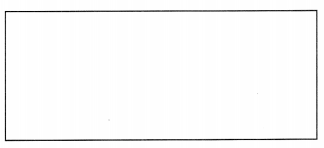I have _______ stickers.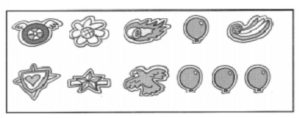Write about the number of stickers everyone has. Fill in the blanks with the correct names.

Question 3.
____ has more stickers than _____________
Pete has more stickers than Ty.

Question 4.
________ has fewer stickers than _______
Ty has fewer stickers than Pete

Question 5.
_____________ has the greatest number of stickers.
Pete has the greatest number of stickers.

Question 6.
_____________ has the least number of stickers.
Ty has the least number of stickers.

Challenging Practice

Question 1.
Class 1A of Greenfield School holds a basketball contest. Find out who won.
CLUES
Rita scores the least number of baskets. John scores 3 more baskets than Rita. Dion scores more baskets than Rachel but less than Frank.

Write the names next to the number of baskets scored.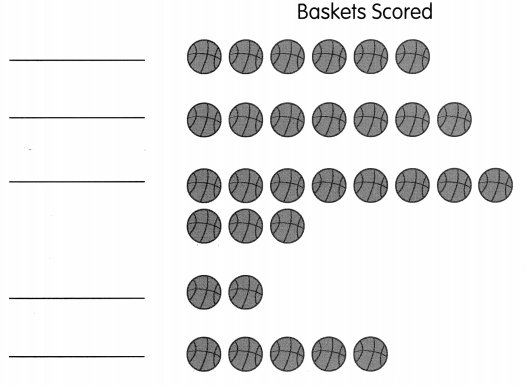Who won the contest? ____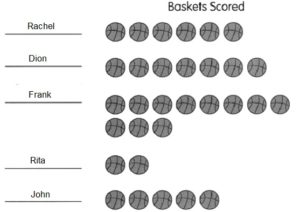Fill in the blanks.

Question 2.
10 + ___ = 15
10 + 5 = 15

Question 3.
10 + ___ = 11
10 + 1 = 11

Question 4.
10 + ___ = 18
10 + 8 = 18

Question 5.
___ + 10 = 14
4 + 10 = 14

Question 6.
___ + 10 = 17
7 + 10 = 17

Write the correct names.

Question 7.
These are the numbers of 12 players on a team.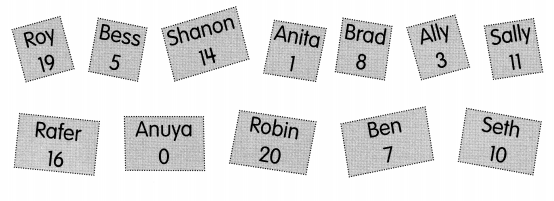Whose names have the following numbers?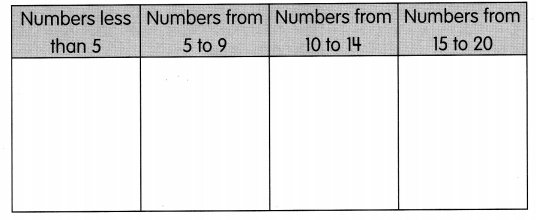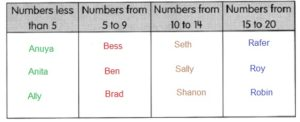Problem Solving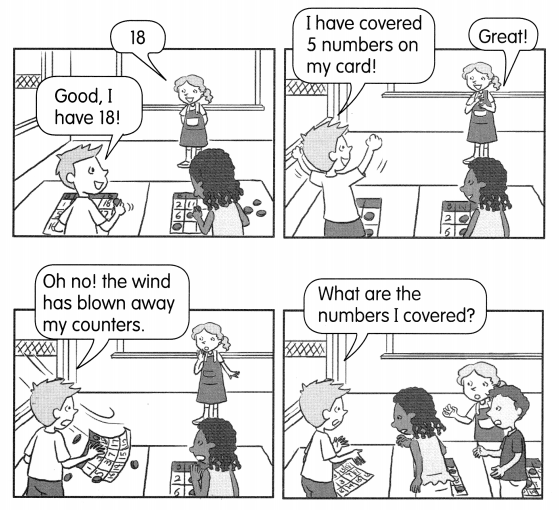Use the clues on the next page. Help Tony find which numbers his counters covered.

Circle the numbers that were covered on Tony’s card.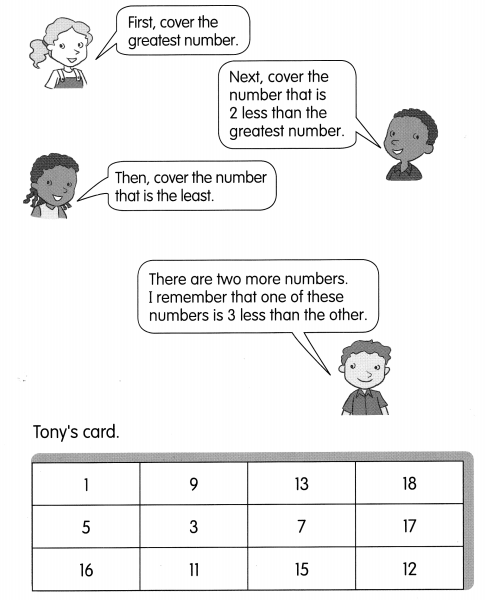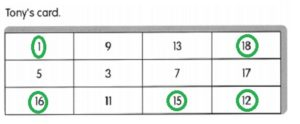Chapter Review/Test

Vocabulary

Unscramble the letters to spell each number.

Question 1.
15 fieetfn
_______
Fifteen

Question 2.
11 evleen
________
Eleven

Question 3.
18 egeethni
_______
Eighteen

Question 4.
20 twynet
_____________
Twenty

Fill in the blank with the correct word.

place-value chart compare

Question 5.
You can show numbers as tens and ones in a _______
You can show numbers as tens and ones in a place-value chart

Question 6.
When you ____ 12 and 15,12 is the number that is less.
When you Compare 12 and 15,12 is the number that is less.

Concepts and Skills

Count. Write the number.

Question 7.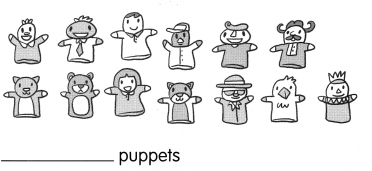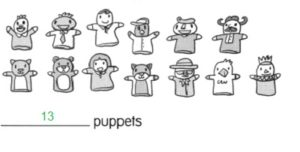Question 8.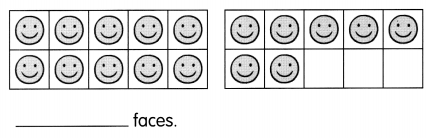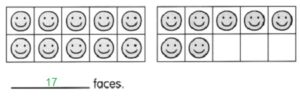Fill in the blanks.

Question 9.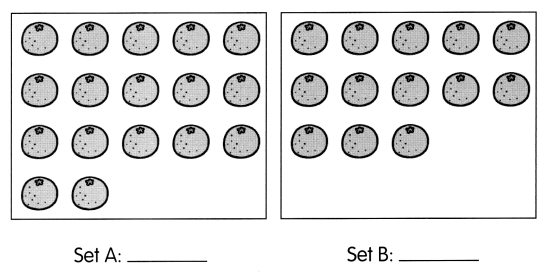Set ___ has ___ more oranges than Set ____
Set ___ has ___ fewer oranges than Set ____.
Which is the greater number? ____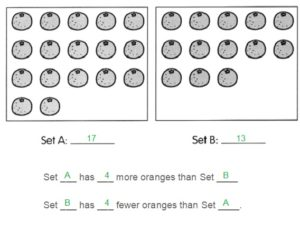Complete the number pattern.

Question 10.
13, 14, ___, ____, 17, ___, 19
Answer: 13, 14, 15, 16, 17, 18, 19

Question 11.
19, 17, ___, 13, 11, ___
19, 17, 15, 13, 11, 9

Write the numbers in order from least to greatest.

Question 12.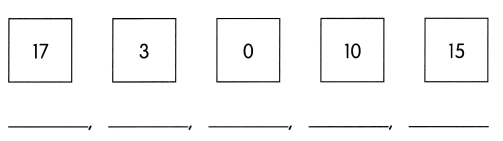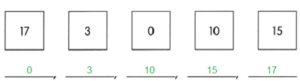Write the numbers in order from greatest to least.

Question 13.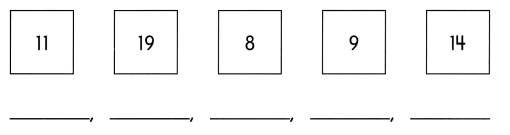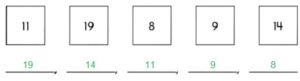Problem Solving
Then cross out the numbers to solve.

Example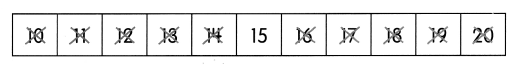I am greater than 13.
I am less than 17.
Of the numbers that are left:
I am not the least.
I am not the greatest.
What number am I? 15

Question 14.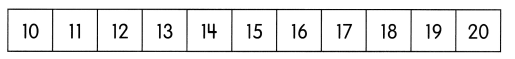I am less than 20.
I am more than 13.
I am less than 17.
I am 4 more than 12.
What number am I? _______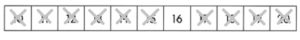Question 15.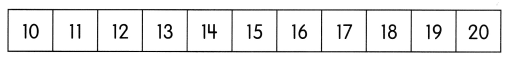a. I am more than 10.
I am less than 20.
I am more than 12.
I am less than 15.
Of the numbers that are left: I am the greater number. What number am I? ____